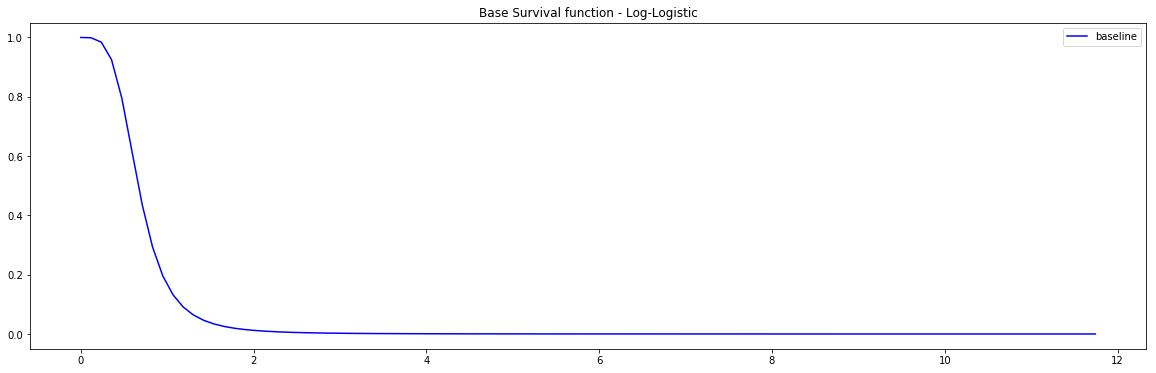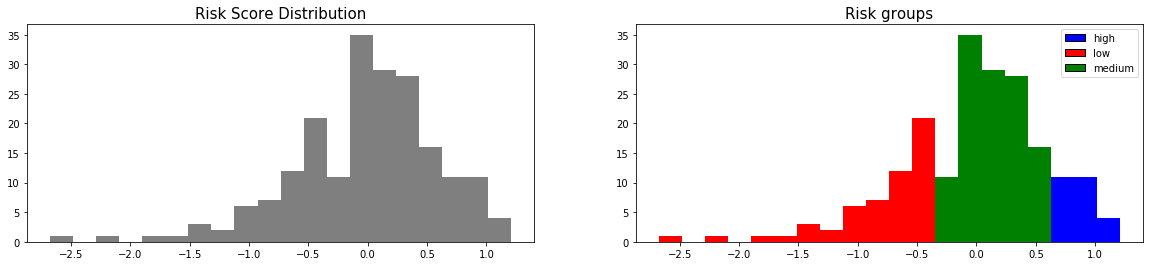# Linear SVM model

The Linear SVM model available in PySurvival is an adaptation of the work of Sebastian Polsterl et al..

## Instance

To create an instance, use pysurvival.models.svm.LinearSVMModel.

## Methods

fit - Fit the estimator based on the given parameters

fit(X, T, E, with_bias = True, init_method='glorot_normal', lr = 1e-2,
max_iter = 100, l2_reg = 1e-4, tol = 1e-3, verbose = True)


Parameters:

• X : array-like -- input samples; where the rows correspond to an individual sample and the columns represent the features (shape=[n_samples, n_features]).

• T : array-like -- target values describing the time when the event of interest or censoring occurred.

• E : array-like -- values that indicate if the event of interest occurred i.e.: E[i]=1 corresponds to an event, and E[i] = 0 means censoring, for all i.

• with_bias: bool (default=True) -- whether a bias should be added

• init_method : str (default = 'glorot_uniform') -- initialization method to use. Here are the possible options:

• glorot_uniform: Glorot/Xavier uniform initializer
• he_uniform: He uniform variance scaling initializer
• uniform: Initializing tensors with uniform (-1, 1) distribution
• glorot_normal: Glorot normal initializer,
• he_normal: He normal initializer.
• normal: Initializing tensors with standard normal distribution
• ones: Initializing tensors to 1
• zeros: Initializing tensors to 0
• orthogonal: Initializing tensors with a orthogonal matrix,
• lr: float (default=1e-4) -- learning rate used in the optimization

• max_iter: int (default=100) -- maximum number of iterations in the Newton optimization

• l2_reg: float (default=1e-4) -- L2 regularization parameter for the model coefficients

• alpha: float (default=0.95) -- confidence level

• tol: float (default=1e-3) -- tolerance for stopping criteria

• verbose: bool (default=True) -- whether or not producing detailed logging about the modeling

Returns:

• self : object

predict_risk - Predicts the risk score $r(x)$

predict_risk(x, use_log=True)


Parameters:

• x : array-like -- input samples; where the rows correspond to an individual sample and the columns represent the features (shape=[n_samples, n_features]). x should not be standardized before, the model will take care of it

• use_log: bool (default=False) -- whether or not appliying the log function to the risk values

Returns:

• risk_score: numpy.ndarray -- array-like representing the prediction of the risk score

## Example

Let's now see how to use the LinearSVMModel models on a simulation dataset generated from a parametric model.

#### 1 - Importing packages
import numpy as np
import pandas as pd
from matplotlib import pyplot as plt
from pysurvival.models.svm import LinearSVMModel
from pysurvival.models.simulations import SimulationModel
from pysurvival.utils.metrics import concordance_index
from sklearn.model_selection import train_test_split
from scipy.stats.stats import pearsonr
# %pylab inline # to use in jupyter notebooks

#### 2 - Generating the dataset from the parametric model
# Initializing the simulation model
sim = SimulationModel( survival_distribution = 'Log-Logistic',
risk_type = 'linear',
censored_parameter = 1.1,
alpha = 1.5, beta = 4)

# Generating N Random samples
N = 1000
dataset = sim.generate_data(num_samples = N, num_features = 4)

# Showing a few data-points


We can now see an overview of the data:

x_1 x_2 x_3 x_4 time event
113.0 15.526830 0.002320 116.0 6.3 0.
118.0 5.293601 0.005194 110.0.0 0.0 0.

Pysurvival also displays the Base Survival function of the Simulation model:

from pysurvival.utils.display import display_baseline_simulations
display_baseline_simulations(sim, figure_size=(20, 6))Figure 1 - Base Survival function of the Simulation model

#### 3 - Splitting the dataset into training and testing sets
# Defining the features
features = sim.features

# Building training and testing sets #
index_train, index_test = train_test_split( range(N), test_size = 0.2)
data_train = dataset.loc[index_train].reset_index( drop = True )
data_test  = dataset.loc[index_test].reset_index( drop = True )

# Creating the X, T and E input
X_train, X_test = data_train[features], data_test[features]
T_train, T_test = data_train['time'].values, data_test['time'].values
E_train, E_test = data_train['event'].values, data_test['event'].values

#### 4 - Creating an instance of the Linear SVM model and fitting the data.
svm_model = LinearSVMModel()
svm_model.fit(X_train, T_train, E_train, init_method='he_uniform',
with_bias = True, lr = 0.5,  tol = 1e-3,  l2_reg = 1e-3)

#### 5 - Cross Validation / Model Performances
c_index = concordance_index(svm_model, X_test, T_test, E_test) #0.93
print('C-index: {:.2f}'.format(c_index))


Because we cannot predict a survival function with LinearSVMModel, let's look at the risk scores and see how correlated they are to the actual risk scores generated from the Simulation model.

#### 6 - Comparing the model predictions to Actual risk score
# Comparing risk scores
svm_risks = svm_model.predict_risk(X_test)
actual_risks = sim.predict_risk(X_test).flatten()
print("corr={:.4f}, p_value={:.5f}".format(*pearsonr(svm_risks, actual_risks)))
# corr=-0.9992, p_value=0.00000


Let's create risk groups based on the risk score distributions

from pysurvival.utils.display import create_risk_groups

risk_groups = create_risk_groups(model=svm_model, X=X_test,
use_log = True,  num_bins=20,  figure_size=(20, 4),
low={'lower_bound':-3.5, 'upper_bound':-0.5, 'color':'red'},
medium={'lower_bound':-0.5, 'upper_bound':0.5,'color':'green'},
high={'lower_bound':0.5, 'upper_bound':2.1,  'color':'blue'}
)Figure 2 - Creating risk groups